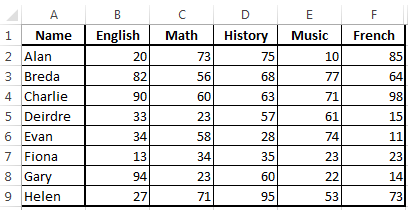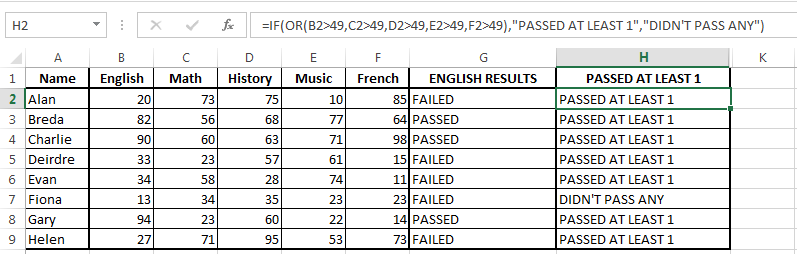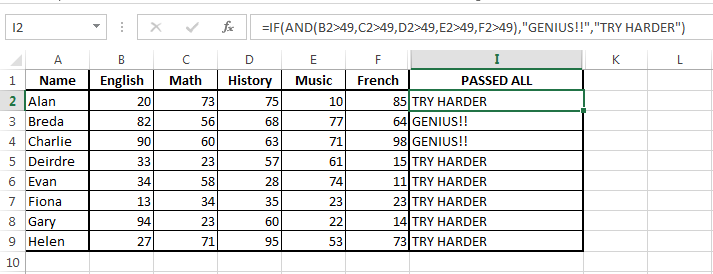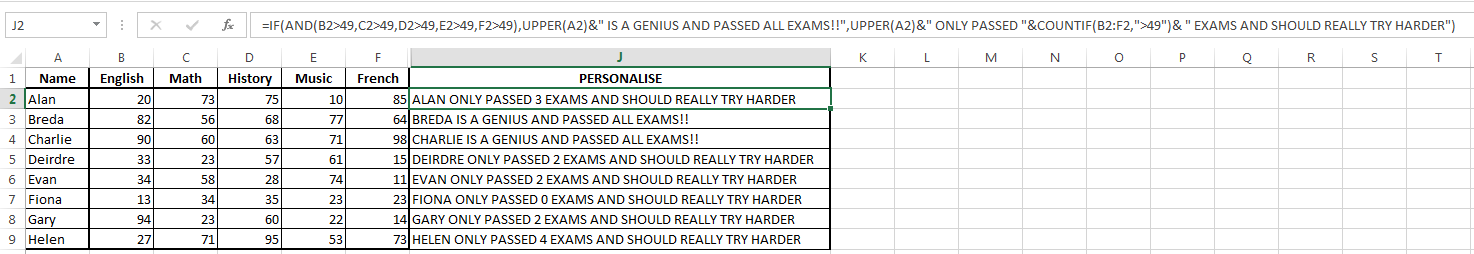# IF Statements

IF statements are usually thought relatively early in Excel courses soon after addition, subtraction, multiplication, etc.  For this reason I guess a lot of people consider them to be “basic” formulas.  However, you would probably be surprised by the number of questions I get about IF statements.  Some people don’t fully understand them or find them too long and confusing.  Personally I think they just find these formulas daunting to look at – without trying to break them down.

See my earlier post here which talks about brackets and commas – very important characters when entering formulas and especially when breaking down formulas.

Do you understand this formula?

=IF(AND(B6>49,C6>49,D6>49,E6>49,F6>49),UPPER(A6)&” IS A GENIUS AND PASSED ALL EXAMS!!”,UPPER(A6)&” ONLY PASSED “&COUNTIF(B6:F6,”>49″)& ” EXAMS AND SHOULD REALLY TRY HARDER”)

If you don’t understand it, ask yourself is it because you don’t understand the formula involved?  Is it too long? Are there too many formulas within formulas?  Is it too daunting to even try to break down?

In this post I will go through IF, IF AND and IF OR statements, break them down and then hopefully the above formula will make perfect sense to you!  While you are reading this post just remember to use the brackets and formulas – pay attention to them and the formulas are easier to understand!

The last section of this post (IF Statement Break Down) will break down the above statement and explain it to you.

The table below lists exam scores for each person listed.  This table will be used to explain the different IF statements.  The pass rate for an exam is 50%# IF Statements

An IF Statement, according to Excel is made up of 3 parts : Logical test, Value if true, Value if false.

In our example, we are going to test if each person passed the English exam.

In plain english our formula is going to be

• IF the results for english are greater than 49*
• THEN say “PASSED”
• Or ELSE say “FAILED”

When I was first learning formulas the IF-THEN-ELSE statement is how we were thought it and that is still how I remember it today.

*The pass rate is 50, so any value greater than 49 is a pass.  The IF statement could also state if the result is greater than or equal to 50 – that would also work.

For Alan, the formula will be entered in cell G2 and his exam result is in cell B2.  Using his results the formula is :

=IF(A2>49, “PASSED”,”FAILED”)

Putting this into english we are saying IF the value in A2 is greater than 49 THEN say “PASSED” Or ELSE say “FAILED”.  Note that the commas separate each of the three parts of the statement.  Without them the formula won’t work because Excel won’t know what the test, value if true or value if false are.

That is how simple an IF statement is – I still use IF-THEN-ELSE to put together the statement and it works for me.The IF AND and IF OR statements will build on this basic IF Statement.

## IF OR

The IF OR statement allows you to test for different logical tests.  The IF statement is still made up of 3 parts : Logical test, Value if true, Value if false.

The difference here is that the logical test is made up of more than one logical tests.

The full make up of the formula is now IF(OR(LogicalTest1, LogicalTest2, LogicalTest3,etc), value if true, value if false).

With our example, we are going to test if the student passed at least 1 exam.  In plain english we are testing IF the student passed english, OR Math, OR History, OR Music OR French THEN say “PASSED AT LEAST 1” Or ELSE say “DIDN’T PASS ANY”.

We start our IF statement as normal, but the logical test begins with OR( and there is a comma between each test before closing with a closing bracket, ).

• Logical Test : OR(B2>49, C2>49, D2>49, E2>49, F2>49)
• Value if True : “PASSED AT LEAST ONE”
• Value if False : “DIDN’T PASS ANY”

Note again the importance of brackets – The whole IF statement is contained in the outer brackets and the OR tests are contained within brackets within the IF brackets.

Commas separate each OR test and also each part of the overall IF Statement.  Use the brackets and commas to break down the formula.

Hopefully at this point, you understand why I am using an IF OR statement here.  The full formula is

=IF(OR(B2>49,C2>49,D2>49,E2>49,F2>49),”PASSED AT LEAST 1″,”DIDN’T PASS ANY”)The IF OR formula may look longer and more daunting than the simple IF statement but as I said already (and I’ll say many more times) pay attention to the brackets and commas to break down the formula – that is how you will understand the formula.

## IF AND

The IF AND statement is similar to the IF OR statement except the IF OR statement is testing if any of the logical tests are true.  The IF AND statement is testing if all the logical tests are true.

In our case, IF Alan passed English AND Math AND History AND Music AND French, THEN he is a “GENIUS!!” Or ELSE he SHOULD “TRY HARDER”.

The IF statement is still made up of 3 parts : Logical test, Value if true, Value if false.  This time, the logical test will again be made up of more than 1 logical test, but here we are testing if all tests are true.

The full make up of the formula is now IF(AND(LogicalTest1, LogicalTest2, LogicalTest3,etc), value if true, value if false).

In our case, IF Alan passed English AND Math AND History AND Music AND French, THEN he is a “GENIUS!!” Or ELSE he SHOULD “TRY HARDER”.

We start our IF statement as normal, but the logical test begins with AND( and there is a comma between each test before closing with a closing bracket, ).

• Logical Test : AND(B2>49, C2>49, D2>49, E2>49, F2>49)
• Value if True : “GENIUS!!”
• Value if False : “TRY HARDER”

Commas separate each AND test and also each part of the overall IF Statement.  I don’t usually like repeating myself so much but – use the brackets and commas to break down the formula.

Hopefully at this point, you understand why I am using an IF AND statement here.  The full formula is

=IF(AND(B2>49,C2>49,D2>49,E2>49,F2>49),”GENIUS!!”,”TRY HARDER”)You can probably guess what I’m about to say……. pay attention to the brackets and commas, they will help you to break down the formula (which by now I’m hoping is looking less daunting).

## IF Statement Break Down

The formula we began with was:

=IF(AND(B6>49,C6>49,D6>49,E6>49,F6>49),UPPER(A6)&” IS A GENIUS AND PASSED ALL EXAMS!!”,UPPER(A6)&” ONLY PASSED “&COUNTIF(B6:F6,”>49″)& ” EXAMS AND SHOULD REALLY TRY HARDER”)

Does it make sense to you yet?  Probably not because I have thrown in a couple more pieces but hopefully you have taken a minute to try to break it down for yourself – that’s a start!

Let’s break down the formula by each comma

• =IF(AND : We are using an IF AND Statement
• (B6>49,C6>49,D6>49,E6>49,F6>49) : Our logical test if testing if all subjects have been passed (the same as the previous IF AND statement).
•  UPPER(A6)&” IS A GENIUS AND PASSED ALL EXAMS!!” : Even though this looks different, it is still nothing more than our value if the logical tests are all true.  The & and UPPER sections are briefly covered below.
• UPPER(A6)&” ONLY PASSED “&COUNTIF(B6:F6,”>49″)& ” EXAMS AND SHOULD REALLY TRY HARDER” : Again, as different as this looks it is still nothing more than our value if the logical tests are not all true (value if false).  Note that this is not the value if all our tests are false – it is the value if not all our logical tests are true.  It may seem like a small difference, but it is an important difference to note.  If the student did not pass all exams, then we want to say that they only passed X number of exams and should really try harder.

### UPPER

In our example, this is just a formatting formula.  UPPER(A6) takes the value in cell A6 and makes it all upper case – taking “Alan” and giving us “ALAN”.

### &

I use the & formula as a starting point when giving some of my classes.  See this post that covers it more.The & parts of our formula allow us to piece together different values to make one string of values.  In our first formula it is taking the value in A6 and then added “IS A GENIUS AND PASSED ALL EXAMS!!”.

A6&” IS A GENIUS AND PASSED ALL EXAMS!!” would give us – “Alan IS A GENIUS AND PASSED ALL EXAMS!!”.  I use the UPPER formula to change Alan to ALAN.  I could have set up the table with the names in capitals, or set up the formula to give “is a genius and passed all exams!!” in lower case and both would have looked OK – but I wanted to give you a bit of a fright with the longer formula!

### COUNTIF

The COUNTIF formula will be covered in more detail in future posts because it deserves more explanation.

For now, it is enough to say that the COUNTIF formula is made up of 2 parts : Range, Criteria.

The Range is the area that you want to look in for your criteria, for Alan this is between cells B6 and F6.  In our formula our criteria is a pass mark (>49) and we want to add up how many times the student has passed an exam.

### Back to the IF Statement Break Down

Using Alan and looking at the formula again we can see:

• =IF(AND(B6>49,C6>49,D6>49,E6>49,F6>49) :  Testing IF Alan passed all exams
•  UPPER(A6)&” IS A GENIUS AND PASSED ALL EXAMS!!” : If he did pass all exams(which he didn’t), THEN return “ALAN IS A GENIUS AND PASSED ALL EXAMS!!” – note the name is now in upper case because of the UPPER formula.
• UPPER(A6)&” ONLY PASSED “&COUNTIF(B6:F6,”>49″)& ” EXAMS AND SHOULD REALLY TRY HARDER” : Or ELSE return “ALAN ONLY PASSED 3 EXAMS AND SHOULD REALLY TRY HARDER”Within our IF Statement we have an AND statement, UPPER, COUNTIF and &.  But none of these changed their format because they are part of a bigger formula.

Here it comes!….. pay attention to the commas and brackets and you should be able to break down the formula and understand it.

In our case, the IF AND statement is the same as any IF AND statement, the COUNTIF, UPPER and & formulas are still the same as if they were used on their own, the only difference here is that they are used as part of a bigger formula.  The commas and brackets are still needed and in the same places.